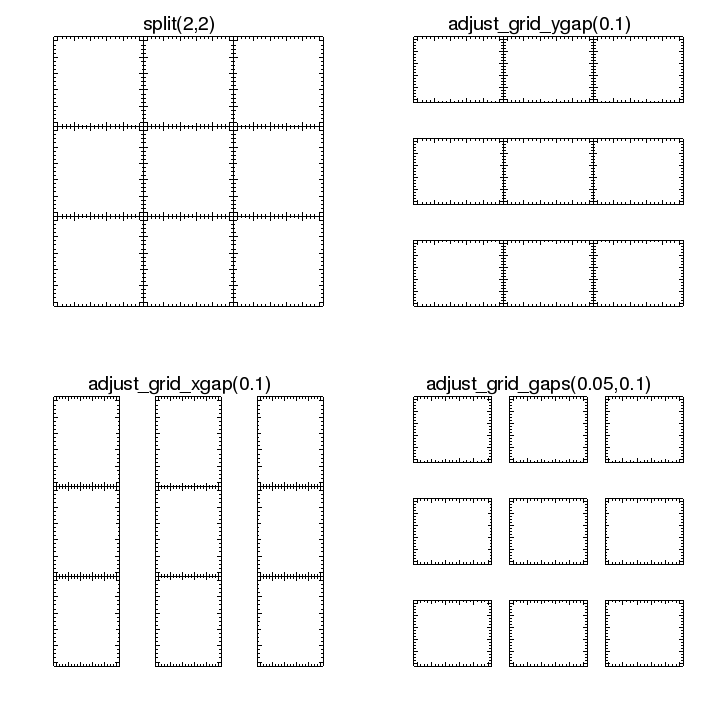## Can I change the spacing between plots?

If you have created a grid of plots using either strip_chart or split then you can use the adjust_grid_gaps set of commands to change the horizontal or vertical spacing between the plots.

The spacing is measured in the frame-normalized coordinate system, so 0.1 represents ten percent of the width or height of the frame containing the plots.

### Change the vertical space between plots

chips> adjust_grid_ygap(0.1)

### Change the horizontal space between plots

chips> adjust_grid_xgap(0.1)

### Change both horizontal and vertical spaces between plots

chips> adjust_grid_gaps(0.05, 0.1)

The following visualization shows the effect of these three commands on the grid of plots created by a split(3,3) call.